# R/lineup2 User Guide

R/lineup2 is an R package providing tools for detecting and correcting sample mix-ups between two sets of measurements, such as between gene expression data on two tissues. It’s a revised version of lineup, to be more general and not so closely tied to the R/qtl package.

We will illustrate some of the tools in this User Guide. We will use the example data included in the package lineup2ex. These are a subset of data from Broman et al. (2015) (see also Tian et al. 2015), and concern gene expression microarray data on about 500 mice in two tissues, gastrocnemius muscle (abbreviated “gastroc”) and pancreatic islets (abbreviated “islet”). While the original data included 40,000 gene expression measurements, the example data consider just a subset of 200 genes: 100 chosen to be highly correlated between the two tissues, and 100 others chosen at random.

When we load the lineup2 package, the data are immediately available, as lineup2ex, which is a list containing the two tissues, each of which is a matrix, samples × genes.

library(lineup2)
gastroc <- lineup2ex$gastroc islet <- lineup2ex$islet

We seek to identify sample mix-ups: mislabeled rows in one or the other data sets. One approach to do this is to first identify a subset of correlated columns, and then to look at the correlations between rows, for that subset of columns.

### Aligning rows or columns

To calculate the correlations between columns, we first need to align the rows between the two matrices. We can do this with the function align_matrix_rows(). There is a similar function align_matrix_cols() for aligning columns.

aligned <- align_matrix_rows(gastroc, islet)

The original matrices have 498 and 499 rows. The output of align_matrix_rows() is a list with two matrices, where the original matrices are reduced to their common rows, based on their row names. The rows are also placed in the same order. Here, each matrix in the output has 497 rows, which is the number of samples in common between the two tissues.

We could use align_matrix_cols() to align the columns (subsetting to the common column names and putting them in the same order), but here they are already aligned, and so no change occurs.

aligned <- align_matrix_cols(aligned[], aligned[])

### Correlations between matrices

With the rows now aligned, we can now calculate the correlation in gene expression between the two tissues, to identify genes that will be useful for measuring sample similarity.

The function corr_betw_matrices() is used to calculate the correlation between the columns in one matrix and the columns in another matrix. There are several options; here we will use what="paired" to get just the correlations between the paired columns.

paired_corr <- corr_betw_matrices(aligned[], aligned[])

The result is a vector of 200 correlations, for the pairs of columns. Here is a plot of the sorted correlations.

par(mar=c(4.1, 5.1, 1.1, 1.1), las=1)
plot(sort(paired_corr), ylab="Correlations between column pairs, sorted", pch=16)
abline(h=0, lty=2, col="gray60")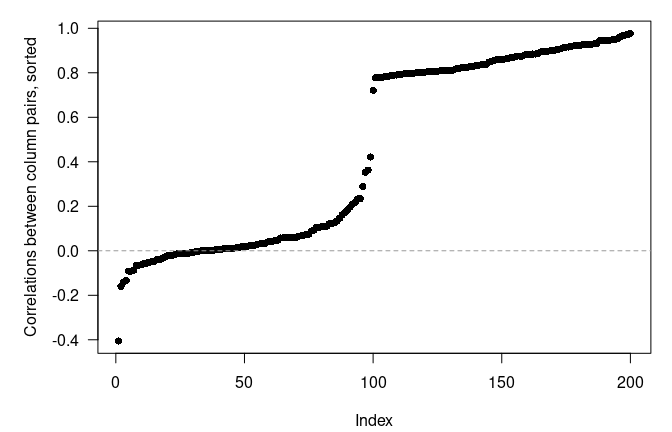Because of the way the genes were selected, there are 100 genes with between-tissue correlation scattered around 0, and then another 100 genes with correlations > 0.75. We will grab that top 100 for assessing sample similarity.

selected_genes <- which(paired_corr > 0.75)

### Correlations/distances between samples

We can now calculate the similarity between the samples using these selected genes. The simplest way to do so is by again using corr_betw_matrices(), but with the transposed matrices so that the samples become the columns.

corr_betw_samples <- corr_betw_matrices(t(gastroc[,selected_genes]),
t(islet[,selected_genes]), what="all")

The output is a 498 × 499 matrix, with the (i,j)th element being the correlation between the ith row of gastroc and the jth row of islet.

Note that there is a related function dist_betw_matrices() to calculate the Euclidean or mean absolute distance between samples. There is also dist_betw_arrays() for arrays with >2 dimensions. Unlike corr_betw_matrices(), these functions look for distance between rows not columns.

### Summarize correlations/distances

The first thing to do with the resulting similarity matrix is to plot the values. The function hist_self_nonself() plots histograms of the self-self correlations and the self-nonself correlations.

hist_self_nonself(corr_betw_samples, xlabel="correlation")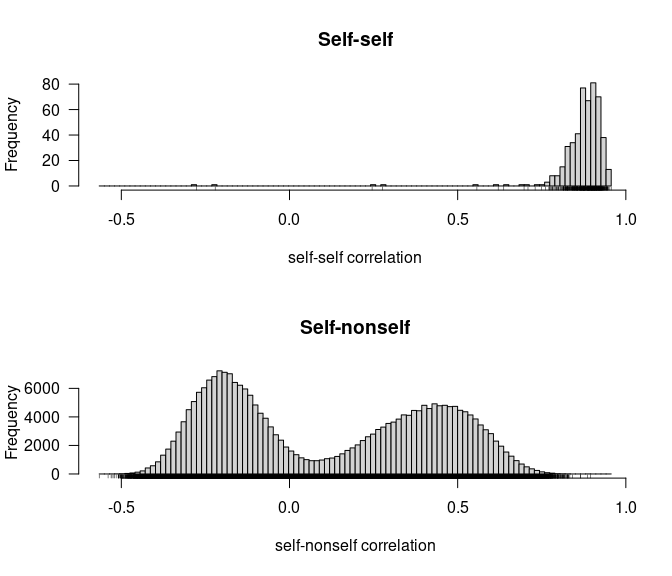The self-self correlations are mostly > 0.7, while the self-nonself correlations are mostly < 0.7. The bimodal distribution of the self-nonself correlations is due to sex differences. Same-sex mice generally show positive correlations, while opposite-sex mice generally show negative correlations.

### Identify sample mix-ups

There are a few self-self correlations that are small and even negative. These are the sample mix-ups we are looking for. To identify the problem samples, we want to pull out the individual values, and identify the maximum values in each row and column.

You can pull out the self-self correlations with the function get_self(). These are the cases where the row and column names match.

self <- get_self(corr_betw_samples)

There is also a function get_nonself() for pulling out the non-self values.

Note that there are 3 missing values, where a sample is present in only one of the two tissues:

self[is.na(self)]
## Mouse3475 Mouse3325 Mouse3666
##        NA        NA        NA

Here are the smallest eight values. As we will see, the first five of these are the real problems.

sort(self)[1:8]
## Mouse3655 Mouse3659 Mouse3598 Mouse3599 Mouse3296 Mouse3538 Mouse3353 Mouse3144
##    -0.277    -0.221     0.246     0.277     0.557     0.620     0.637     0.697

The samples with low self-self correlation are likely mix-ups. To identify the correct labels, we can look for the largest correlation in each row and column of the similarity matrix. To do this, we use the function get_best(). We use the argument get_min=FALSE since we are looking for the maximum here, and we use the argument dimension to control whether to look within each row (gastroc) or within each column (islet).

best_byrow <- get_best(corr_betw_samples, get_min=FALSE, dimension="row")
best_bycol <- get_best(corr_betw_samples, get_min=FALSE, dimension="column")

We can plot the self-self correlations against the maximum correlations by row and column. (There is a bit of effort to get labels on the points.)

par(mfrow=c(1,2), mar=c(4.1, 4.1, 1.6, 0.6), las=1)
plot(self, best_byrow, xlab="Self-self correlation", ylab="Best islet correlation",
main="Gastroc samples", pch=16, xlim=c(-0.3, 1.0), ylim=c(0.6, 1.0))
label <- best_byrow-self > 0.2
text(self[label]+0.03, best_byrow[label], names(self)[label],

plot(self, best_bycol, xlab="Self-self correlation", ylab="Best gastroc correlation",
main="Islet samples", pch=16, xlim=c(-0.3, 1.0), ylim=c(0.6, 1.0))
label <- best_bycol-self > 0.3
text(self[label]+0.03, best_bycol[label], names(self)[label],
label <- best_bycol-self > 0.2 & best_bycol-self < 0.3
text(self[label]-0.03, best_bycol[label], names(self)[label],
adj=c(1,0.5), cex=0.8)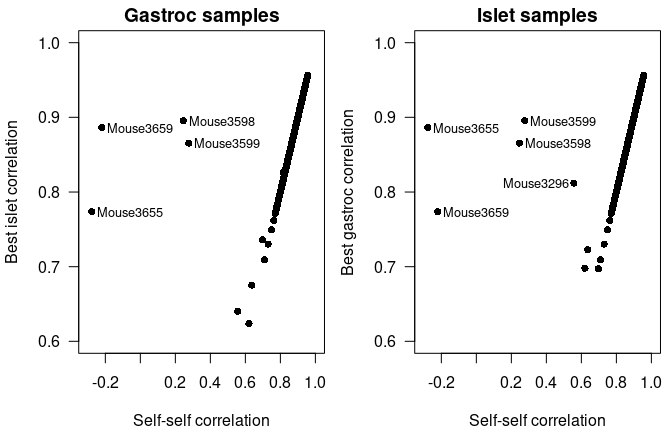Most samples have a large self-self correlation that matches the maximum in the row and column. There are four gastroc samples that have low self-self correlation but where there is another large value in that row, and there are five problem islet samples.

The function get_problems() can be used to get a summary of potential problems. Looking by row or by column, you can pull out the samples where the best distance exceeds the self-self distance by more than some threshold.

Looking by row, and using a threshold of 0.2, we see these samples:

get_problems(corr_betw_samples, threshold=0.2, get_min=FALSE)
##         ind   self  best which_best next_best which_next_best
## 1 Mouse3659 -0.221 0.886  Mouse3655     0.716       Mouse3161
## 2 Mouse3655 -0.277 0.774  Mouse3659     0.593       Mouse3309
## 3 Mouse3598  0.246 0.896  Mouse3599     0.776       Mouse3539
## 4 Mouse3599  0.277 0.866  Mouse3598     0.750       Mouse3193
## 5 Mouse3475     NA 0.713  Mouse3649     0.699       Mouse3432

This shows the four problems in the left panel of the above figure, and that they constitute two apparent sample swaps (Mouse3655 ↔︎ Mouse3659 and Mouse3598 ↔︎ Mouse3599).

Looking by column, again using a threshold of 0.2, we see the same results plus one more:

get_problems(corr_betw_samples, threshold=0.2, get_min=FALSE, dimension="column")
##         ind   self  best which_best next_best which_next_best
## 1 Mouse3655 -0.277 0.886  Mouse3659     0.691       Mouse3248
## 2 Mouse3659 -0.221 0.774  Mouse3655     0.656       Mouse3558
## 3 Mouse3598  0.246 0.866  Mouse3599     0.704       Mouse3469
## 4 Mouse3599  0.277 0.896  Mouse3598     0.758       Mouse3285
## 5 Mouse3296  0.557 0.812  Mouse3295     0.626       Mouse3648
## 6 Mouse3325     NA 0.824  Mouse3142     0.787       Mouse3488
## 7 Mouse3666     NA 0.698  Mouse3354     0.694       Mouse3121

We again see the Mouse3655 ↔︎ Mouse3659 and Mouse3598 ↔︎ Mouse3599 pairs, and also see that Mouse3296 sample in islet looks to correspond to Mouse3295 (gastroc).

Note that the function which_best() can be used to provide the sample IDs that correspond to the results of get_best(); sort of like which.max() vs max(). This is another way to see that islet Mouse3296 looks like Mouse3295.

which_best(corr_betw_samples, get_min=FALSE, dimension="column")["Mouse3296"]
##   Mouse3296
## "Mouse3295"

Another way to look at these problems is to plot the values in each column. We can use the plot_sample() function, indicating dimension="column" (versus the default, by row), and get_min=FALSE since we’re interested in the maximum correlation (versus the minimum distance). The most similar sample is indicated in red, while the self sample is indicated in green.

samples <- sort(c(names(self)[best_bycol-self > 0.2], "Mouse3295"))
par(mfrow=c(3,2), las=1, mar=c(4.1, 4.1, 2.1, 0.6))
for(sample in samples) {
plot_sample(corr_betw_samples, sample, "column", get_min=FALSE)
}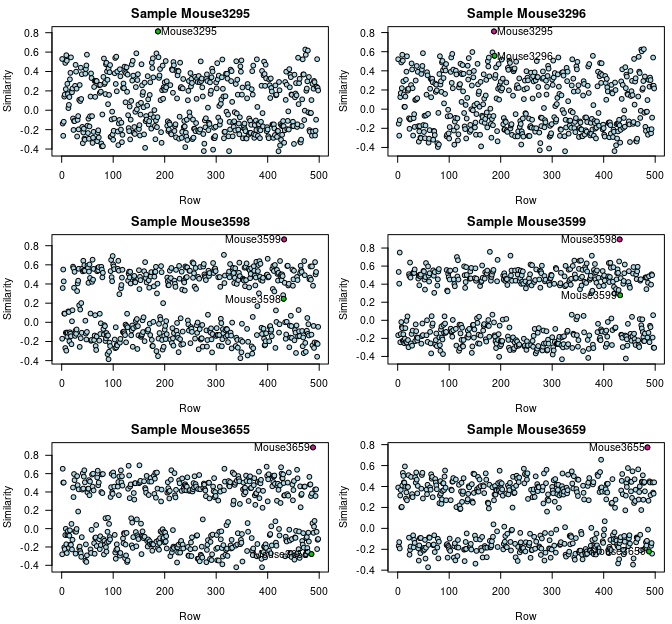From these results, we can see that Mouse3598 and Mouse3599 look to be switched in one or the other tissue, as are Mouse3655 and Mouse3659. From these data on their own, we can’t tell which tissue is the problem, but the original data include gene expression microarrays for four other tissues, and by comparisons to those tissues, it turns out that Mouse3655/Mouse3659 were mixed up in gastroc, while Mouse3598/Mouse3599 were mixed up in islet. See Broman et al. (2015), Fig. 4.

Samples Mouse3295 and Mouse3296 look correct in gastroc, and Mouse3295 looks correct in islet, but Mouse3296 islet looks like Mouse3295 gastroc. The sample labelled Mouse3296 in islet is really an unintended biological replicate of sample Mouse3295.

### Second-best samples

As further support to show that the best-correlated samples correspond to the correct labels, it can be useful to consider the second-best sample in each row and column. If the best-correlated sample is not much more correlated than the second-best, than the support is not so strong, for the best sample to be the true one.

To pull out the second-best correlated sample, use the function get_2ndbest(), which has the same arguments as get_best().

secbest_byrow <- get_2ndbest(corr_betw_samples, get_min=FALSE, dimension="row")
secbest_bycol <- get_2ndbest(corr_betw_samples, get_min=FALSE, dimension="column")

We then can plot the second-best samples versus the best samples, and we will point to the problem samples. The four problem samples in gastroc, and the five problem samples in islet, are highlighted in red.

red <- "#ff4136"
par(mfrow=c(1,2), mar=c(4.1, 4.1, 1.6, 0.6), las=1)
plot(best_byrow, secbest_byrow, xlab="Best islet correlation",
ylab="Second best islet correlation",
main="Gastroc samples", pch=16, xlim=c(0.5, 1), ylim=c(0.5, 1))
label <- best_byrow-self > 0.2
points(best_byrow[label], secbest_byrow[label], pch=16, col=red)
abline(0,1, lty=2)

plot(best_bycol, secbest_bycol, xlab="Best gastroc correlation",
ylab="Second best gastroc correlation",
main="Islet samples", pch=16, xlim=c(0.5, 1), ylim=c(0.5, 1))
label <- best_bycol-self > 0.2
points(best_bycol[label], secbest_bycol[label], pch=16, col=red)
abline(0,1, lty=2)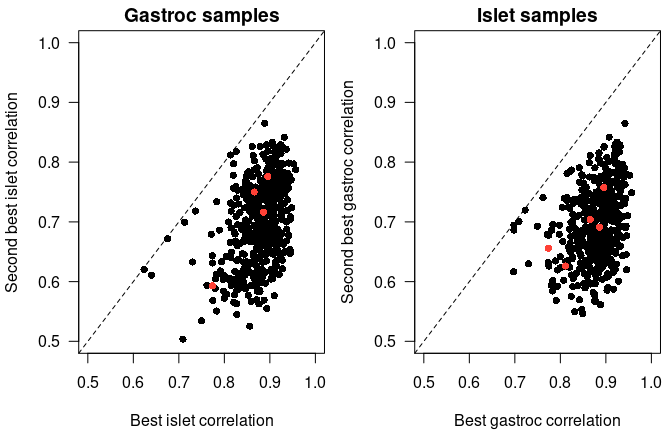Values away from the diagonal have good support for the “best” sample being the correct one. This includes all of the problem samples (in red).

Note that there is which_2ndbest() function, similar to which_best() but pulling out the second-closest sample.